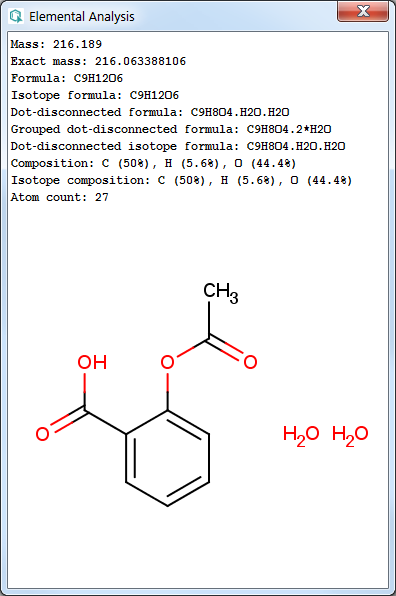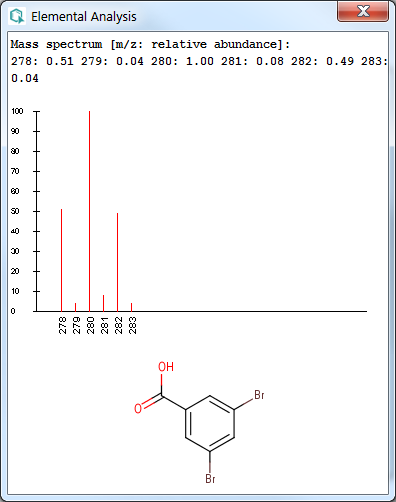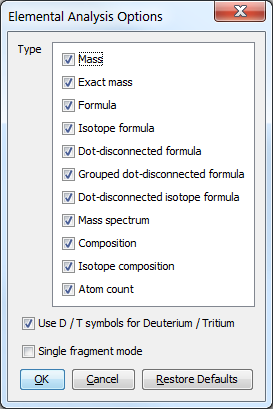# Elemental Analysis Plugin

This manual gives you a walk-through on how to use the Elemental Analysis Plugin:

# Introduction

The Elemental Analysis Plugin calculates basic descriptors related to the elemental composition of a molecule.

The example below illustrates the analysis results for an input structure.Fig. 1 Elemental Analysis result window examples

# Options

In the Elemental Analysis Options window you can set the following options:

• Type

1. Mass: average molecular mass calculated from the standard atomic weights.

2. Exact mass: monoisotopic mass calculated from the weights of the most abundant natural isotopes of the elements.

3. Formula: chemical formula of the molecule according to the Hill system: the number of carbon atoms is indicated first, the number of hydrogen atoms next, and then the number of all other chemical elements subsequently, in alphabetical order. Isotopes (like Deuterium and Tritium) are not listed separately but counted together (e.g., deuterium and tritium atoms are counted as hydrogens). When the formula contains no carbon, all the elements, including hydrogen, are listed alphabetically. If the molecule contains an SRU or Repeating Unit S-group, it is taken into account and Polymer Formula is generated.

4. Isotope formula: gives the chemical formula of the molecule with separately listed isotopes, according to the Hill system.

5. Dot-disconnected formula: chemical formula of the molecule, with fragment formulae separated by dots (e.g. salts, counterions, solvent molecules).

6. Grouped dot-disconnected formula: chemical formula of the molecule, with the fragment formulae separated by dots and multiple fragments grouped together with the number of repetition

7. Dot-disconnected isotope formula: chemical formula of the molecule, with the fragment formulae separated by dots and separately listed isotopes.

8. Mass spectrum: calculates the isotope distribution of the input molecule(s). The result is displayed as a spectrum, which plots the mass/charge values (m/z) vs. the relative abundance.

9. Composition: elemental composition given in weight percentage (w/w %), calculated from the atomic masses.

10. Isotope composition: elemental composition with separately listed isotopes (w/w %).

11. Atom count: number of all atoms in the molecule.

For polymers mass, composition and atom count calculations are not available. NaN, N/A, and -1 values are returned in these cases, respectively.

• Use D/T symbols for Deuterium/Tritium: if unchecked (default), isotopes of hydrogen are displayed as 2H and 3H; otherwise D and T symbols are used.

• Single fragment mode: if unchecked (default), the calculation handles unlinked molecules together (e.g. salt molecules), summing up the masses of each component; otherwise the results are displayed in a scroll window.Fig. 2 Elemental Analysis Options window

# References

1. Atom weights: M. E. Wieser, "Atomic weights of the elements 2005 (IUPAC Technical Report)" Pure Appl. Chem., Vol. 78, No. 11, pp. 2051-2066, 2006; doi

2. Isotope weights: G.Audi and A.H.Wapstra, "The 1995 update to the atomic mass evaluation" Nuclear Physics A595 vol. 4, pp. 409-480, 1995;doi

3. The Hill system: E. A. Hill, "On A System Of Indexing Chemical Literature; Adopted By The Classification Division Of The U. S. Patent Office". J. Am. Chem. Soc., 22(8), pp. 478-494, 1900; doi